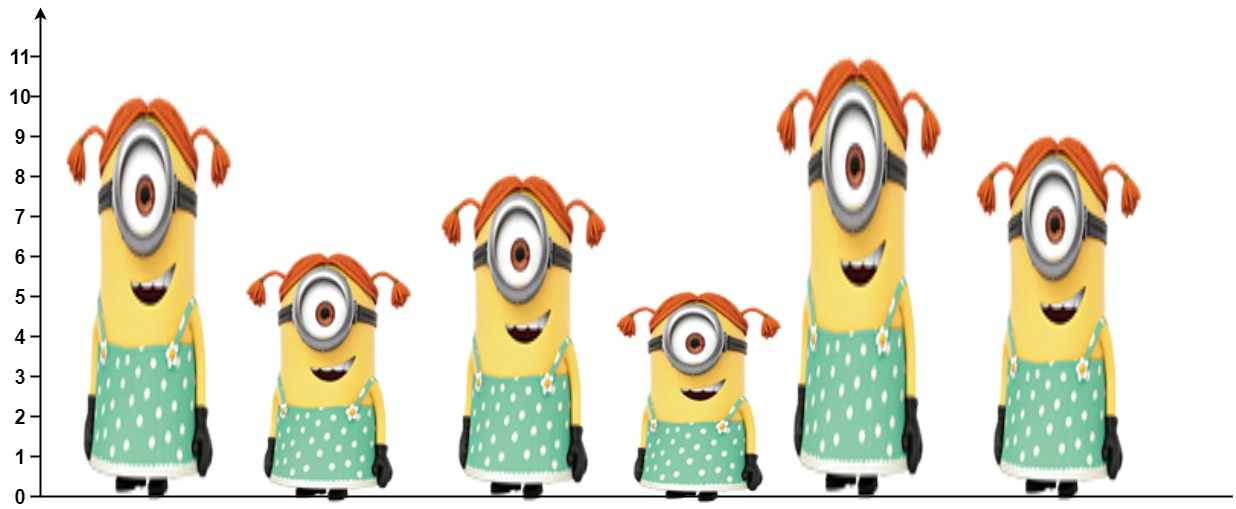##### Welcome to Subscribe On Youtube

Formatted question description: https://leetcode.ca/all/1944.html

# 1944. Number of Visible People in a Queue

Hard

## Description

There are n people standing in a queue, and they numbered from 0 to n - 1 in left to right order. You are given an array heights of distinct integers where heights[i] represents the height of the i-th person.

A person can see another person to their right in the queue if everybody in between is shorter than both of them. More formally, the i-th person can see the j-th person if i < j and min(heights[i], heights[j]) > max(heights[i+1], heights[i+2], ..., heights[j-1]).

Return an array answer of length n where answer[i] is the number of people the i-th person can see to their right in the queue.

Example 1:Input: heights = [10,6,8,5,11,9]

Output: [3,1,2,1,1,0]

Explanation:

Person 0 can see person 1, 2, and 4.

Person 1 can see person 2.

Person 2 can see person 3 and 4.

Person 3 can see person 4.

Person 4 can see person 5.

Person 5 can see no one since nobody is to the right of them.

Example 2:

Input: heights = [5,1,2,3,10]

Output: [4,1,1,1,0]

Constraints:

• n == heights.length
• 1 <= n <= 10^5
• 1 <= heights[i] <= 10^5
• All the values of heights are unique.

## Solution

Use monotonic stack. Loop over heights backward. For each height heights[i], count the number of people that are already in the stack and have heights less than the current height, and pop the elements from the stack until the stack becomes empty or the top element of the stack is strictly greater than the current height, and push heights[i] into the stack. The value of answer[i] is the number of popped elements before pushing heights[i], and if the stack is not empty when stopping popping elements, add 1 to answer[i]. Finally, return answer.

• class Solution {
public int[] canSeePersonsCount(int[] heights) {
int length = heights.length;
int[] counts = new int[length];
for (int i = length - 1; i >= 0; i--) {
int height = heights[i];
while (!stack.isEmpty()) {
int prevHeight = stack.peek();
counts[i]++;
if (prevHeight <= height)
stack.pop();
else
break;
}
stack.push(height);
}
return counts;
}
}

• // OJ: https://leetcode.com/problems/number-of-visible-people-in-a-queue/
// Time: O(NlogN)
// Space: O(N)
class Solution {
public:
vector<int> canSeePersonsCount(vector<int>& A) {
vector<int> ans(A.size());
deque<int> q;
int N = A.size();
for (int i = N - 1; i >= 0; --i) {
int h = A[i];
auto it = lower_bound(begin(q), end(q), h);
if (it != end(q) && *it > h) ++ans[i];
ans[i] += it - begin(q);
while (q.size() && q.front() <= h) q.pop_front();
q.push_front(h);
}
return ans;
}
};

• class Solution:
def canSeePersonsCount(self, heights: List[int]) -> List[int]:
n = len(heights)
ans =  * n
stack = list()

for i in range(n - 1, -1, -1):
while stack:
ans[i] += 1
if heights[i] > stack[-1]:
stack.pop()
else:
break
stack.append(heights[i])

return ans

############

# 1944. Number of Visible People in a Queue
# https://leetcode.com/problems/number-of-visible-people-in-a-queue/

from sortedcontainers import SortedList
​
class Solution:
def canSeePersonsCount(self, heights: List[int]) -> List[int]:
n = len(heights)
res =  * (n - 1) + 
sl = SortedList()
mp = collections.defaultdict(int)

for i, h in list(enumerate(heights))[::-1]:
index = sl.bisect_left(h)
​
if sl:
res[i] = max(1, min(len(sl), index + 1))

if i + 1 < n and h > heights[i + 1]:
for _ in range(index):
sl.pop(0)